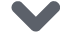# PyTorch专栏（七）:模型保存与加载那些事

### 本文是全系列中第12 / 24篇：Pytorch 专栏【磐创AI 导读】：本篇文章讲解了PyTorch专栏的第三章中的保存和加载模型查看专栏历史文章，请点击下方蓝色字体进入相应链接阅读。查看关于本专栏的介绍：PyTorch专栏开篇。想要更多电子杂志的机器学习，深度学习资源，大家欢迎点击上方蓝字关注我们的公众号：磐创AI

• PyTorch简介
• PyTorch环境搭建

• 微调基于torchvision 0.3的目标检测模型
• 微调TorchVision模型
• 空间变换器网络
• 使用PyTorch进行神经传递
• 生成对抗示例
• 使用ONNX将模型转移至Caffe2和移动端

• 聊天机器人教程
• 使用字符级RNN生成名字
• 使用字符级RNN进行名字分类
• 在深度学习和NLP中使用Pytorch
• 使用Sequence2Sequence网络和注意力进行翻译

1. torch.save将序列化对象保存到磁盘。此函数使用Python的pickle模块进行序列化。使用此函数可以保存如模型、tensor、字典等各种对象。

2. torch.load使用pickle的unpickling功能将pickle对象文件反序列化到内存。此功能还可以有助于设备加载数据。

3. torch.nn.Module.load_state_dict使用反序列化函数 state_dict 来加载模型的参数字典。

## 1.什么是状态字典：state_dict?

# 定义模型class TheModelClass(nn.Module):    def __init__(self):        super(TheModelClass, self).__init__()        self.conv1 = nn.Conv2d(3, 6, 5)        self.pool = nn.MaxPool2d(2, 2)        self.conv2 = nn.Conv2d(6, 16, 5)        self.fc1 = nn.Linear(16 * 5 * 5, 120)        self.fc2 = nn.Linear(120, 84)        self.fc3 = nn.Linear(84, 10)    def forward(self, x):        x = self.pool(F.relu(self.conv1(x)))        x = self.pool(F.relu(self.conv2(x)))        x = x.view(-1, 16 * 5 * 5)        x = F.relu(self.fc1(x))        x = F.relu(self.fc2(x))        x = self.fc3(x)        return x# 初始化模型model = TheModelClass()# 初始化优化器optimizer = optim.SGD(model.parameters(), lr=0.001, momentum=0.9)# 打印模型的状态字典print("Model's state_dict:")for param_tensor in model.state_dict():    print(param_tensor, "t", model.state_dict()[param_tensor].size())# 打印优化器的状态字典print("Optimizer's state_dict:")for var_name in optimizer.state_dict():    print(var_name, "t", optimizer.state_dict()[var_name])
• 输出

Model's state_dict:conv1.weight     torch.Size([6, 3, 5, 5])conv1.bias   torch.Size()conv2.weight     torch.Size([16, 6, 5, 5])conv2.bias   torch.Size()fc1.weight   torch.Size([120, 400])fc1.bias     torch.Size()fc2.weight   torch.Size([84, 120])fc2.bias     torch.Size()fc3.weight   torch.Size([10, 84])fc3.bias     torch.Size()Optimizer's state_dict:state    {}param_groups     [{'lr': 0.001, 'momentum': 0.9, 'dampening': 0, 'weight_decay': 0, 'nesterov': False, 'params': [4675713712, 4675713784, 4675714000, 4675714072, 4675714216, 4675714288, 4675714432, 4675714504, 4675714648, 4675714720]}]

## 2.保存和加载推理模型

### 2.1 保存/加载state_dict（推荐使用）

• 保存

torch.save(model.state_dict(), PATH)
• 加载

model = TheModelClass(*args, **kwargs)model.load_state_dict(torch.load(PATH))model.eval()

• 注意

load_state_dict()函数只接受字典对象，而不是保存对象的路径。这就意味着在你传给load_state_dict()函数之前，你必须反序列化 你保存的state_dict例如，你无法通过 model.load_state_dict(PATH)来加载模型。

### 2.2 保存/加载完整模型

• 保存

torch.save(model, PATH)
• 加载

# 模型类必须在此之前被定义model = torch.load(PATH)model.eval()

## 3. 保存和加载 Checkpoint 用于推理/继续训练

• 保存

torch.save({            'epoch': epoch,            'model_state_dict': model.state_dict(),            'optimizer_state_dict': optimizer.state_dict(),            'loss': loss,            ...            }, PATH)
• 加载

model = TheModelClass(*args, **kwargs)optimizer = TheOptimizerClass(*args, **kwargs)checkpoint = torch.load(PATH)model.load_state_dict(checkpoint['model_state_dict'])optimizer.load_state_dict(checkpoint['optimizer_state_dict'])epoch = checkpoint['epoch']loss = checkpoint['loss']model.eval()# - or -model.train()

## 4. 在一个文件中保存多个模型

• 保存

torch.save({            'modelA_state_dict': modelA.state_dict(),            'modelB_state_dict': modelB.state_dict(),            'optimizerA_state_dict': optimizerA.state_dict(),            'optimizerB_state_dict': optimizerB.state_dict(),            ...            }, PATH)
• 加载

modelA = TheModelAClass(*args, **kwargs)modelB = TheModelBClass(*args, **kwargs)optimizerA = TheOptimizerAClass(*args, **kwargs)optimizerB = TheOptimizerBClass(*args, **kwargs)checkpoint = torch.load(PATH)modelA.load_state_dict(checkpoint['modelA_state_dict'])modelB.load_state_dict(checkpoint['modelB_state_dict'])optimizerA.load_state_dict(checkpoint['optimizerA_state_dict'])optimizerB.load_state_dict(checkpoint['optimizerB_state_dict'])modelA.eval()modelB.eval()# - or -modelA.train()modelB.train()

PyTorch 中常见的保存 checkpoint 是使用 .tar 文件扩展名。

## 5. 使用在不同模型参数下的热启动模式

• 保存

torch.save(modelA.state_dict(), PATH)
• 加载

modelB = TheModelBClass(*args, **kwargs)modelB.load_state_dict(torch.load(PATH), strict=False)

## 6. 通过设备保存/加载模型

### 6.1 保存到 CPU、加载到 CPU

• 保存

torch.save(model.state_dict(), PATH)
• 加载

device = torch.device('cpu')model = TheModelClass(*args, **kwargs)model.load_state_dict(torch.load(PATH, map_location=device))

### 6.2 保存到 GPU、加载到 GPU

• 保存

torch.save(model.state_dict(), PATH)
• 加载

device = torch.device("cuda")model = TheModelClass(*args, **kwargs)model.load_state_dict(torch.load(PATH))model.to(device)# 确保在你提供给模型的任何输入张量上调用input = input.to(device)

### 6.3 保存到 CPU，加载到 GPU

• 保存

torch.save(model.state_dict(), PATH)
• 加载

device = torch.device("cuda")model = TheModelClass(*args, **kwargs)model.load_state_dict(torch.load(PATH, map_location="cuda:0"))  # Choose whatever GPU device number you wantmodel.to(device)# 确保在你提供给模型的任何输入张量上调用input = input.to(device)

### 6.4 保存 torch.nn.DataParallel 模型

• 保存

torch.save(model.module.state_dict(), PATH)
• 加载

# 加载任何你想要的设备

torch.nn.DataParallel是一个模型封装，支持并行GPU使用。要普通保存 DataParallel 模型, 请保存model.module.state_dict()` 这样，你就可以非常灵活地以任何方式加载模型到你想要的设备中。

●  PyTorch专栏（六）: 混合前端的seq2seq模型部署
●  PyTorch专栏（一）
●下方点击 |   | 了解更多